# July 2003

Research:
Dances of the Planets
by Howard Arrington

The planets in the heavens move in exquisite orbital patterns, dancing to the Music of the Cosmos.  There is more mathematical and geometric harmony than we realize.   The idea for this article is from a book Larry Pesavento shared with me.  The book, 'A Little Book of Coincidence' by John Martineau, illustrates the orbital patterns and several of their geometrical relationships.  .

Take the orbits of any two planets and draw a line between the two planet positions every few days.  Because the inner planet orbits faster than the outer planet, interesting patterns evolve.  Each planetary pairing has its own unique dance rhythm.  For example, the Earth-Venus dance returns to the original starting position after eight Earth years.  Eight Earth years equals thirteen Venus years.  Note that 8 and 13 are members of the Fibonacci number series.

• Earth:     8 years * 365.256 days/year  =  2,922.05 days
• Venus:  13 years * 224.701 days/year  =  2,921.11 days (ie. 99.9%)

Watching the Earth-Venus dance for eight years creates this beautiful five-petal flower with the Sun at the center.  (5 is another Fibonacci number.)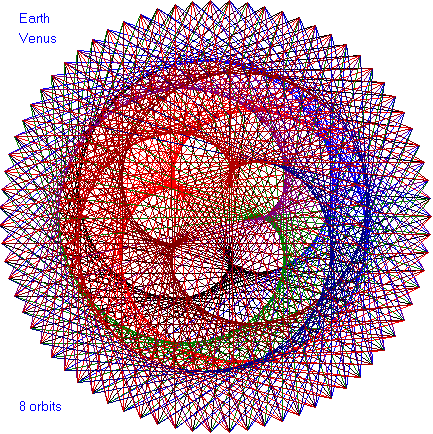Another intriguing fact is the ratio between the Earth's outer orbit and Venus's inner orbit is given by a square.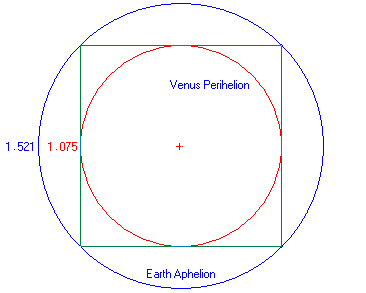In the following dance patterns, the planet pairing is given and the number of orbits of the outer planet.  Enjoy these beautiful patterns.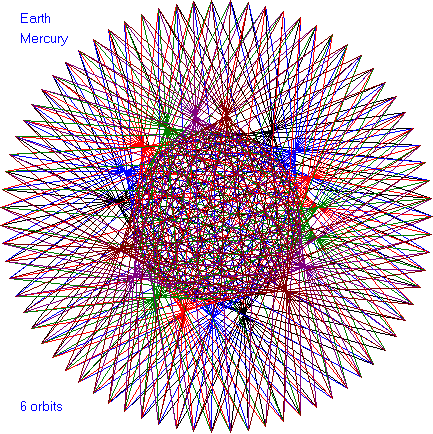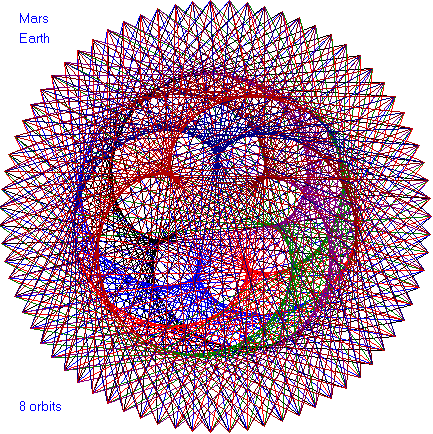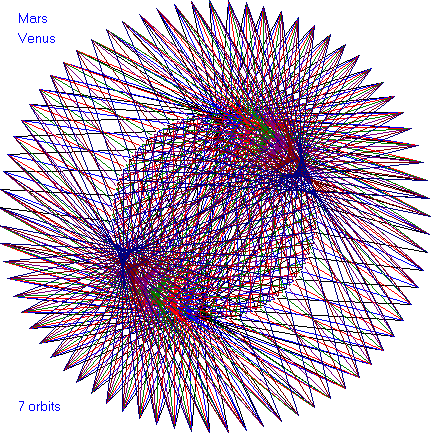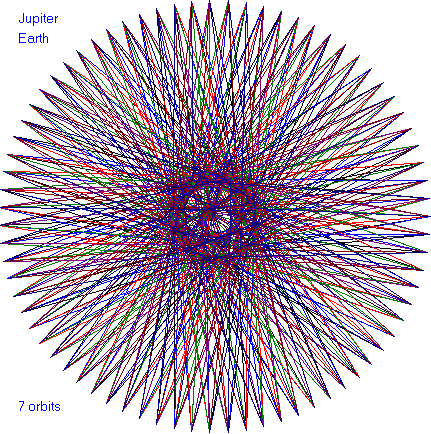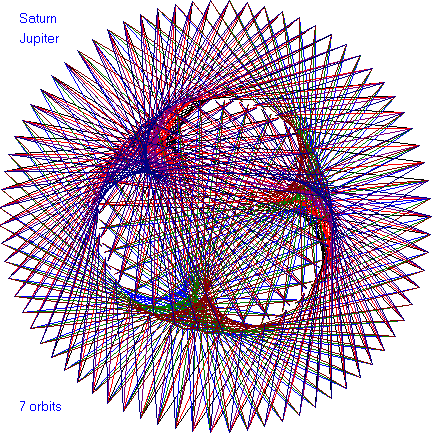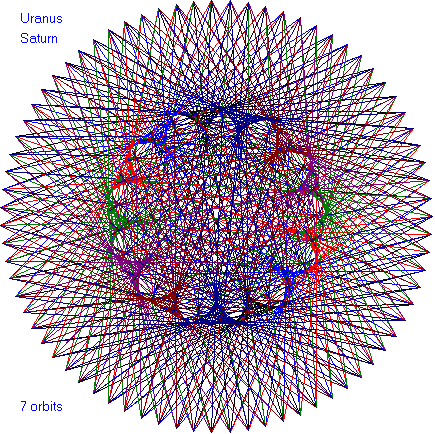Let me share with you other facts about cosmic harmony.  The radius of the Moon compared to the Earth is three to eleven, ie. 3:11.

• Radius of Moon = 1,080 miles =  3 x 360

• Radius of Earth  = 3,960 miles = 11 x 360 = 33 x 1 x 2 x 3 x 4 x 5

• Radius of Earth plus Radius of Moon = 5,040 miles = 1 x 2 x 3 x 4 x 5 x 6 x 7 = 7 x 8 x 9 x 10

The ratio 3:11 is 27.3 percent, and the orbit of the Moon takes 27.3 days.  27.3 days is also the average rotation period of a sunspot.  The closest : farthest distance ratio that Venus and Mars each experiences in the Mars-Venus dance is incredibly 3:11.  The Earth orbits between them.

The sizes of the Moon and the Earth 'Square the Circle' as shown in this illustration, which is drawn to scale.  The perimeters of the dotted square and the dotted circle are the same length.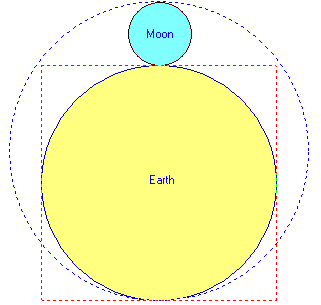The perimeter of the dotted red square is 4 x Earth's diameter = 4 x 7,920 miles = 31,680 miles.
The circumference of the dotted blue circle is 2 pi x radius = 2 x 3.142 x 5040 miles = 31,667 miles.  (ie. 99.9%)

ESPL:
Dances Program
by Howard Arrington

The dance patterns were drawn by the following ESPL program using a chart window for the canvas.  The chart bars and grid were hidden so the canvas was blank.  Edit the Planet1 and Planet2 variable values to select the planet pairing, with the planets numbered beginning with Mercury = 1.

var {global variables}
i,c,Planet1,Planet2: integer;
Planet1Year,Planet2Year: real;
Interval,Orbits: real;
yBottom,yCenter,xCenter: real;
a1,a2,a1Interval,a2Interval: real;
r,r1,r2,rStop: real;
x1,y1,x2,y2: real;

function Year(i: integer): real;  {orbital period in days}
begin
if i=1 then Result:=87.969
else if i=2 then Result:=224.701
else if i=3 then Result:=365.256
else if i=4 then Result:=686.980
else if i=5 then Result:=4332.6
else if i=6 then Result:=10759.2
else if i=7 then Result:=30685
else if i=8 then Result:=60190
else if i=9 then Result:=90465;
end;

function Orbit(i: integer): real;  {mean orbit distance in 10^6 km}
begin
if i=1 then Result:=57.91
else if i=2 then Result:=108.21
else if i=3 then Result:=149.60
else if i=4 then Result:=227.92
else if i=5 then Result:=778.57
else if i=6 then Result:=1433.5
else if i=7 then Result:=2872.46
else if i=8 then Result:=4495.1
else if i=9 then Result:=5869.7;
end;

function Name(i: integer): string;
begin
if i=1 then Result:='Mercury'
else if i=2 then Result:='Venus'
else if i=3 then Result:='Earth'
else if i=4 then Result:='Mars'
else if i=5 then Result:='Jupiter'
else if i=6 then Result:='Saturn'
else if i=7 then Result:='Uranus'
else if i=8 then Result:='Neptune'
else if i=9 then Result:='Pluto';
end;

begin  {main program}
Planet1:= 3; {select outer planet}
Planet2:= 2; {select inner planet}
Orbits := 8; {number of outer rotations}

Planet1Year := Year(Planet1);
Planet2Year := Year(Planet2);
Interval := Planet1Year/75; {days}

FindWindow(eChart);
yBottom:=PriceToY(GetVariable(eScaleLow));
yCenter:=2+yBottom/2;
xCenter:=100+yCenter;

r:=0; rStop:=Planet1Year * Orbits;
a1:=0; a1Interval:=2*pi*Interval/Planet1Year;
a2:=0; a2Interval:=2*pi*Interval/Planet2Year;

SetPen(clBlue);  {print labels}
TextOut(120,10,Name(Planet1));
TextOut(120,30,Name(Planet2));
TextOut(120,yBottom-30,inttostr(Orbits)+' orbits');

while r<rStop do begin
i:=trunc(r/interval/75);  {use different color each orbit}
if i=0 then c:=clBlack
else if i=1 then c:=clBlue
else if i=2 then c:=clRed
else if i=3 then c:=clGreen
else if i=4 then c:=clPurple
else if i=5 then c:=clMaroon
else if i=6 then c:=clNavy
else if i=7 then c:=clDkRed
else c:=clOrange;

SetPen(c,1,eSolid,pmCopy);
a1:=a1-a1Interval; {angle1}
a2:=a2-a2Interval; {angle2}
x1:=r1*Cos(a1); y1:=r1*Sin(a1);  {convert polar to rectangular coordinates}
x2:=r2*Cos(a2); y2:=r2*Sin(a2);

MoveToLineTo(x1+xCenter,y1+yCenter,x2+xCenter,y2+yCenter);  {draw line}
r:=r+Interval;  {move around outer circumference}
end;
end;

Research:
The Art of Optimizing
by Howard Arrington

I recently designed a trading system in Ensign Windows using a few Study Alerts to implement the Buy, Sell, and Exit rules.  The system was simple wherein it used a Parabolic Stop study to determine market direction, and would buy retracements in an uptrend and sell retracements in a downtrend.  The system would exit any position when a scalp objective had been achieved, or when a protective stop was touched.

This trading system had 4 adjustable parameters:   Parabolic Acceleration Rate, Stop Size, Scalp Size, and Retracement Size.   After several quick guesses for each parameter's setting, the test results implied that the system was most sensitive to the parameters in the order as listed.   It seemed logical that the parameters should be optimized in the order of their sensitivity from most sensitive to least sensitive.   Therefore, the first step of optimization was to pick 'in-the-ballpark' parameter values for the other parameters while the Parabolic Acceleration Rate parameter was adjusted in small increments.

The training data set consisted of 10 consecutive days of day session 2-minute bars for the Emini futures contract.   These 10 days included a variety of market conditions such as trending days, sideways choppy days, several large opening gaps up and down, and occasional periods of wild volatility.  The 10-day period seemed sufficient for the purpose of finding appropriate parameter values.  All results are for trading 1 Emini contract with no deduction for commissions or slippage.

To optimize the Parabolic Acceleration Rate, values were adjusted from 0.35 through 0.65 in increments of 0.05 while the other parameters were fixed at these values:      Stop Size = 6 points        Scalp Size = 7 points        Retracement Size = 2 points.     The worst results were \$812 profit on 44 trades using the 0.35 setting.   The best results were \$3887 profit on 49 trades at the 0.50 setting.

The protective Stop Size was optimized by testing values from 1 point through 10 points in 1 point increments.   Scalp Size and Retracement Size remain unchanged.  The Parabolic parameter used the 0.50 setting.  The worst results were \$3337 profit on 54 trades using the 3 point Stop.  The best results were \$3900 on 49 trades using a 7 point Stop.

The Scalp Size was optimized by testing values from 1 point through 10 points in 1 point increments.  The other parameters used were these values:  Retracement Size = 2 points      Stop Size = 7 points        Parabolic = 0.50.     The worst results were \$2312 profit on 100 trades using a 1 point scalp objective.  The best results were \$4500 on 58 trades using a 5 point scalp objective.   This result would lend support to Larry Pesavento's claim that a natural harmonic swing size of 5.40 points exists in the S&P and ES markets.

Finally, the Retracement Size parameter was optimized by testing values from 1 point through 5 points in half point increments.  The worst results were \$62 profit on 5 trades using a 5 point retracement requirement.   The best results were \$4775 profit on 64 trades using a 1.50 point retracement size.

The following example shows the trading system in operation using the optimized parameter values.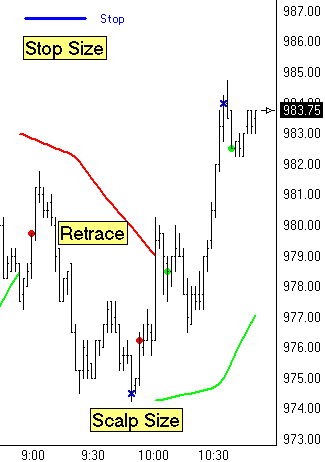Just prior to 9:00 a downward move in the market reversed the Parabolic Stop study to a down trend as indicated by the falling Red curve.   The retrace that followed exceeded the Retracement Size requirement of 1.50 points, so the system sold Short and used as its fill price the Close of the bar marked by the 1st Red dot.   The Scalp objective of 5 points was achieved 50 minutes later so the system exited this Short trade and used as its fill price the Close of the bar marked by the Blue X.   During this Short trade, the protective Stop was 7 points away from the entry price and marked on the chart with a horizontal blue line.

After this successful scalp trade, the retracement requirement was satisfied again while the Parabolic was still in a down trend.  The system sold Short a 2nd time at the 2nd Red dot near 10:00.   The market did not continue lower as expected, but rose to trigger a reversal of the Parabolic Stop to an uptrend indicated by the rising Green curve.  A retracement in the uptrend initiated a Buy signal.  Therefore, the Short trade was closed out at a loss and a Long trade was initiated using as its fill price the Close of the bar marked by the 1st Green dot.   This long trade was successful and existed at the 5 point scalp objective marked by the 2nd Blue X near the swing top.   A 2nd long trade was initiated on the retracement marked by the 2nd Green dot.

Once optimized values were determined for each of the parameters, a quick recheck was done of each of the parameters to see if they had shifted in either direction now that better parameters were known for the others.   The values determined above remained the optimal settings.   Perhaps it was just coincidence, and perhaps it was because the initial 'in-the-ballpark' values were close to the optimized values.   The system has been tuned to optimal performance (at least for the past 10 days) using these values:   Parabolic = 0.50     Stop Size = 7 points     Scalp Size = 5 points     Retracement Size = 1.50 points.  The system was run the next day with 3 successful scalps and 2 losses.  The net for the 5 trades added another \$385 to the total profit.

The next step in the evolution of the system was to examine each of the 64 trades that generated the \$4775 profit in the 10-day trading data set.  The general statistics showed that there were 37 winners (58%) and 27 losers (42%).   While some of the parameter combinations generated win/loss percentages as favorable as 80/20, the profitability was always lower because fewer trades were taken.  For some traders, that would be a desirable characteristic.  For this optimized system the Average Winner was \$180 and the Average Loser was \$70.   Both of these numbers are very good.  The Average Trade Profit was \$75, which is sufficient to pay for a round trip commission and tolerate a tick slippage.

The examination of the trades showed that 7 times the system was in a position at market close and carried the position overnight.  The effect of holding the position overnight was surprisingly favorable with 6 winners and 1 loser with these point totals:  +10, +7, +2, +7, +3, +2, and -7 points for the only loser.  Thus the overnight effect added 24 points or \$1200 to the overall system profitability.  This was an unexpected result, but the 10-day data set included several gap openings, and the system happened to be on the right side of those gaps 6 out of 7 times.

The system was modified to exit any position at market close so no position was held overnight.  This would be a characteristic preferred by day traders, and the overall system performance was less profitable as expected.

The overnight effect was intriguing and generated the need to test that idea as a separate trading system.  So, in a matter of minutes, Study Alerts were configured to Buy the Close of the day session if the Parabolic Stop was Long, and Sell the Close of the day session if the Parabolic Stop was Short.  This simple system would exit on the Close of the first 2 minute bar of the following day's day session open.   That's it.   One trade per day, either buying or selling the last bar of the day session and exiting the following day on the first bar of the next day session.  The system had a \$2300 profit on 9 trades for the 10-days in the test data set.

Now, do any of my ideas and numbers represent the 'Holy Grail' of trading systems.   Absolutely not.  The purpose of this article was to walk system designers through the thought process of how parameters that define a trading system might be adjusted a maximize a system's performance across a test data set.  Some may wish to maximize the win/loss ratio, while others (myself included) prefer to maximize the total dollar profit as long as the trading frequency is not excessive.  For my simple system, 64 trades in 10 days is a trade frequency of 5 to 8 trades per day session, which is realistic for a scalping system.

Here is a example Trade Ledger that logs the details of each of the trades taken by the system.  The form shows some of the statistics used to evaluate whether one set of parameters is better or worse than another set of parameters.  The Notes field shows the Time of day the trade was executed.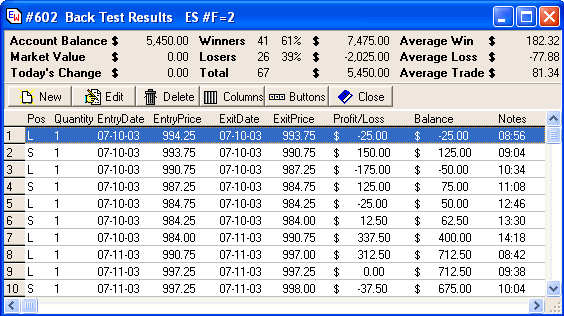Note:  If you have Ensign Windows and wish to experiment with this trading system, it can be downloaded from the Ensign web site using the Internet Services form in Ensign Windows.  Open the Internet Services form and click the Upgrade tab.  Click the Connect button.  Then select the Template bullet and the July24th name in the template list.  Click the Download button to put the system on your computer.  Enjoy.

Disclaimer:  Past performance is no guarantee of equivalent future performance.  All results in this article are hypothetical and do not include commissions and slippage penalties.  This system was optimized for a particular 10-day period of time and designed with the benefit of hindsight.   Results from the system with any other data set, past or future, will in all likelihood be less impressive.  No representation is being made that any account will or is likely to achieve the profits or losses similar to those shown.  This trading system is being shared solely for its educational value and should not be a basis for placing actual trades.